Practice questions available in McGraw Hill Math Grade 2 Answer Key PDF Chapter 3 Test will engage students and is a great way of informal assessment.

Is the number odd or even? Circle your answer. Use small objects to help.

Question 1.
3 odd even
Answer: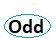3 is an odd number because it is not divisible by 2.

Question 2.
14 odd even
Answer: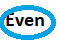14 is an even number because it is divisible by 2 and leaves the remainder 0.

Question 3.
10 odd even
Answer:10 is an even number because it is divisible by 2 and leaves the remainder 0.

Question 4.
17 odd even
Answer:17 is an odd number because it is not divisible by 2.

Question 5.
9 + 10 = ____
odd even
Answer:9 + 10 = 19
So, 19 is an odd number.

Question 6.
11 + 5 = _____
odd even
Answer:11 + 5 = 16
16 is an even number.

Use the pictures to count by 2s. Write the numbers.

Question 7.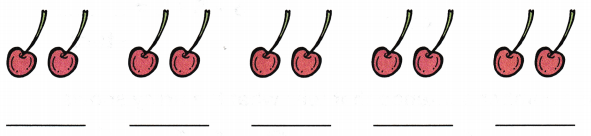Count the number by 2s and find the number of cherries
2 + 2 + 2 + 2 + 2 = 10 cherries

Question 8.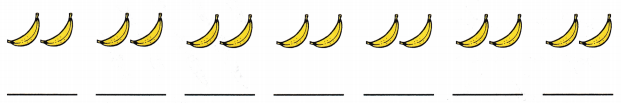Count the bananas by 2s
2 × 7 = 14
Thus there are 14 bananas.

Question 9.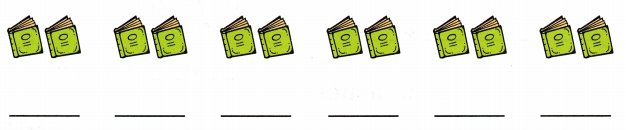Count by 2s
2 + 2 + 2 + 2 + 2 + 2 = 12
6 × 2 = 12

Use the pictures to count by 5s. Write the numbers.

Question 10.Count each group by 5s.
5 + 5 + 5 + 5 = 20

Question 11.Count each group by 5s.
5 + 5 + 5 + 5 + 5 + 5 = 30

Look at the array. Write the sum.

Question 12.3 + 3 + 3 + 3 = _____ cars
There are 4 rows and 3 columns of cars.
3 + 3 + 3 + 3 = 12 cars

Question 13.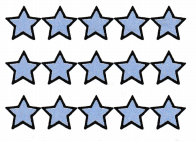5 + 5 + 5 = ____ stars
There are 3 rows and 5 columns of stars
5 + 5 + 5 = 15 stars

Write a number sentence that tells what the array shows.

Question 14.____ + ___ + ____ + ____ = ____ airplanes